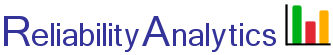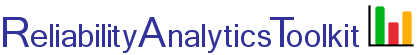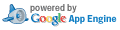# Effective Failure Rate of One Standby Offline Unit with "n" Active On-line Units Required for Success. (with Repair)

This tool calculates the effective failure rate of a configuration that has a single off-line standby unit ("cold spare") with "n" active on-line units required for success. The off-line spare unit is assumed to have a failure rate of zero while it is in a non-operating state. All on-line units are assumed to have equal failure and repair rates. Upon failure of one of the on-line units, the standby off-line unit is switched in with a probability of "P," the probability that that the switching mechanism works properly. For perfect switching, P=1. The calculation implements Equation 3 shown on page 90 of the United States Air Force Rome Laboratory Reliability Engineer's Toolkit (1993). See this page for additional details on time inputs (Mct) for system restoration.

## Reliability Block Diagram: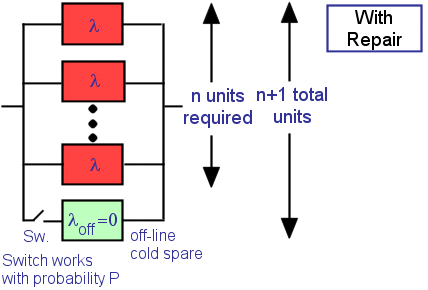## Calculation Inputs:

Number of active on-line units (n):
Mean corrective maintenance time (Mct), hours:
Probability that switch will work correctly (P, range 0 to 1.0):
Decimal places:

## Featured Reference: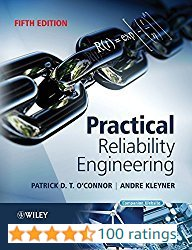Practical Reliability Engineering

Toolkit Home